## Example Questions

### Example Question #1 : How To Find The Length Of The Diagonal Of A Square

If a square has a side length of 4, how long is the diagonal of the square?

8√2

2√2

4√2

4

16

4√2

Explanation:

A diagonal divides a square into two 45-45-90 triangles, which have lengths adhering to the ratio of x: x: x√2. Therefore, 4√2 is the correct answer as the diagonal represents the hypotenuse of the triangle. the Pythagorean theorem can also be used: 42+ 42 = c2.

### Example Question #2 : How To Find The Length Of The Diagonal Of A Square

If a square has a side length of √2, how long is the diagonal of the square?

4√2

2

2√2

4

4-√2

2

Explanation:

A diagonal divides a square into two 45-45-90 triangles, which have lengths adhering to the ratio of x: x: x√2. Therefore, 2 is the correct answer as the diagonal represents the hypotenuse of the triangle. the Pythagorean theorem can also be used: √22+ √22 = c2.

### Example Question #1 : How To Find The Length Of The Diagonal Of A Square

A square has a side of length 5. What is the length of its diagonal?

10

5

10√3

5√3

5√2

5√2

Explanation:

The diagonal separates the square into two 45-45-90 right triangles. The problem can be solved by using the Pythagorean Theorem, a2 + b2 = c2.  It can also be solved by recognizing the 45-45-90 special triangles, which have side ratios of x√2.

### Example Question #4 : How To Find The Length Of The Diagonal Of A Square

A square with width of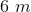is inscribed in a circle. What is the total area inside the circle?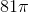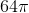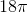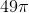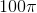Explanation:

We know that each side of the square is 6, so use the Pythagorean Theorem to solve for the diagonal of the square. The diagonal of the square is also the diameter of the circle.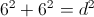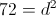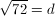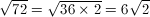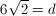Therefore the radius must be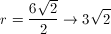Now let's find the area inside the circle using the radius.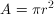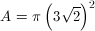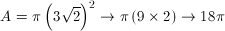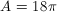meters

### Example Question #5 : How To Find The Length Of The Diagonal Of A Square

Quantity A:

The diagonal of a square with a side-length of.

Quantity B:

The side-length of a square with a diagonal of.

Quantity B is greater.

Quantity A is greater.

The relationship between the two quantities cannot be determined from the information given.

Both quantities are equal.

Both quantities are equal.

Explanation:

Quantity A: The diagonal of a square with a side-length of 7.

Quantity B: The side-length of a square with a diagonal of 14.

Both quantities can be determined, so can the relationship.

Quantity A:

To determine the diagonal of a square, it's important to remember that a diagonal directly bisects a square from corner to corner. In other words, it bisects the corners, creating two triangles with 45:45:90 proportions, with the diagonal serving as the hypotenuses . If you remember your special triangles, then the side-side-hypotenuse measurements have a ratio of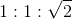In Quantity A, the side-length is 7. Following the proportion: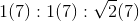The diagonal equals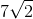.

Quantity B:

We can use the same ratio to figure out quantity B by substituting x for the unknown side-length quantity, which looks like this: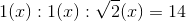To find x in this ratio, just isolate x in the hypotenuse: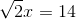Divide by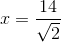Now, how does Quantity A and Quantity B match up?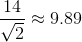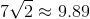On the surface, it looks like the two quanties are equal. But how do we prove it?  Well, we know that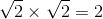.  Therefore, we know that: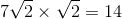Divide both sides by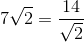Therefore, both quantities are equal.